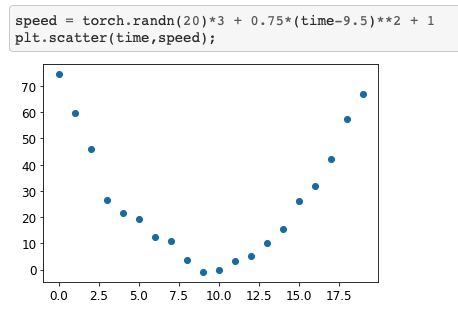# Why author used a quadratic equation for SGD?

In Stochastic Gradient Descent (SGD) section there is example where we want to measure the speed of a roller coaster every second for 20 seconds. Authors use this mathematical equation as the basis:

y = at^2 + bt +c where t = time

My question is why not use a linear equation?

y = mt + b

Hi @arnuld,

You would not be able to fit a “U-shaped” function with a linear equation.

Hope it helps,
Charles

1 Like

Hmm… you mean a parabolic curve relates to variable speed: higher, high, lower, low and zero speed at different times. Speed variation of such type can’t be represented by a straight line. Am I correct?

We are trying to approximate this curve.This can only be done by an equation of order 2 or more. A linear equation only can represent a straight line.

2 Likes

Why we are trying to approximate this curve? Why not approximate a Z or M shape curve? How do we know that a roller-coaster graphs like parabola?It is just a learning example. The point of the exercise is to show that SGD can be used for approximations of curves.
More complicated shapes can be approximated too, and that is literally what deep learning is about (though every input can only produce one output, so Z shapes can not be approximated and your rollercoaster image wouldn’t work either). However, approximating more complicated shapes requires more complicated functions. How this can be done is described in the follow up lectures.

1 Like